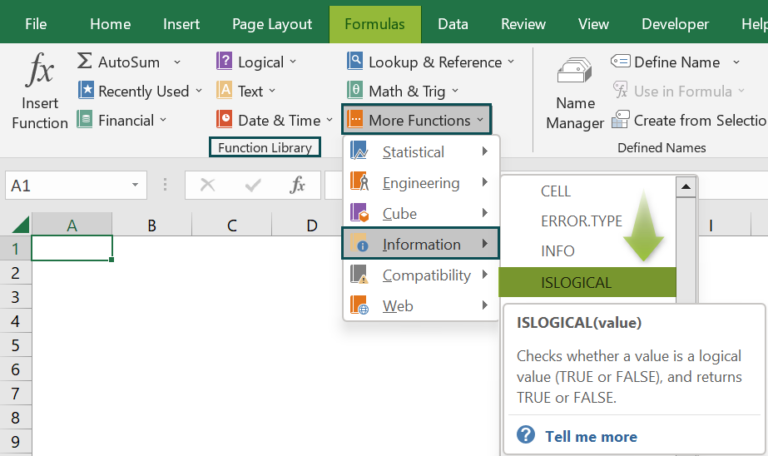# ISLOGICAL Excel FunctionArticle byExcelMojo Team## What Is LOGICAL Function In Excel?

The ISLOGICAL Excel function checks for the logical values, TRUE or FALSE, in a given dataset. If either of the logical values is found, the function returns “TRUE”, else “FALSE”. The ISLOGICAL function in Excel is an inbuilt “Information” function, so we can insert the formula from the “Function Library” or enter it directly in the worksheet.

For example, we will apply the ISLOGICAL function to find the logical values for the given data in column A.

Select cell A2, enter the formula =ISLOGICAL(10), and press “Enter”.

###### Key Takeaways
• The ISLOGICAL Excel function evaluates whether the selected values or the results of the formula cells selected are logical or not.
• Since the function has one mandatory argument, the argument value can be a cell, a cell range, the result of a formula, or the direct value without cell reference.
• The function is mainly used to check for errors while using formulas for calculations during data analysis.
• The function is a part of the IS functions group, such as ISERROR, ISEVEN, ISODD, etc.

### ISLOGICAL() Excel Formula

The syntax of the ISLOGICAL Excel formula is,

The argument of the ISLOGICAL Excel formula is,

• value: It is a mandatory argument. It is a selected cell or a cell range to verify whether it is logical.

### How To Use ISLOGICAL Excel Function?

We can use the ISLOGICAL Excel function in 2 ways, namely,

1. Access from the Excel ribbon.
2. Enter in the worksheet manually.

#### Method #1 – Access from the Excel ribbon

Choose an empty cell for the output → select the “Formulas” tab → go to the “Function Library” group → click the “More Functions” option drop-down → click the “Information” option right arrow → select the “ISLOGICAL” function, as shown below.

The “Function Arguments” window appears. Enter the argument in the “Value” field → click “OK”, as shown below.

#### Method #2 – Enter in the worksheet manually

1. Select an empty cell for the output.
2. Type =ISLOGICAL( in the selected cell. [Alternatively, type =I or =IS and double-click the ISLOGICAL function from the list of suggestions shown by Excel.]
3. Enter the arguments as cell values or cell references in excel and close the brackets.
4. Press the “Enter” key.

We will find the logical value cells using ISLOGICAL Excel function.

In the table, the data is,

• Column A contains the Value.
• Column B contains the Output.

The steps to find the value using ISLOGICAL formula are,

1. Select cell B2, and enter the formula =ISLOGICAL(A2).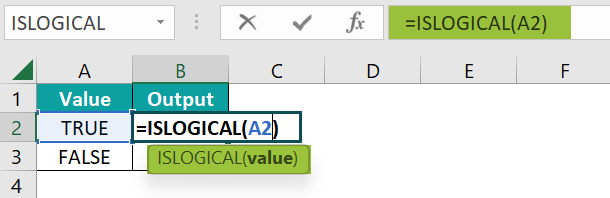2. Press the “Enter” key. The result is “TRUE”, as shown below.3. Drag the formula from cell B2 to B3 using the fill handle.The output is shown above. Since both cells, A2 and A3, have logical values, the results in cells B2 and B3 are TRUE.

### Examples

We will understand some advanced scenarios using the ISLOGICAL Excel function examples.

#### Example #1

We will find the logical value cells for the given numeric values using ISLOGICAL function.

In the table, the data is,

• Column A contains the Value.
• Column B contains the Output.

The steps to find the value using ISLOGICAL formula are,

• Step 1: Select cell B2, enter the formula =ISLOGICAL(A2), and press the “Enter” key. The result is “FALSE”, as shown below.
• Step 2: Drag the formula from cell B2 to B6 using the fill handle. The output is shown below.

#### Example #2

We will find the logical value cells in the given fruit’s data using ISLOGICAL function.

In the table, the data is,

• Column A contains the Value.
• Column B contains the Output.

The steps to find the value using ISLOGICAL formula are,

• Step 1: Select cell B2, enter the formula =ISLOGICAL(A2), and press the “Enter” key. The result is “FALSE”, as shown below.
• Step 2: Drag the formula from cell B2 to B6 using the fill handle. The output is shown below.

#### Example #3

We will find the logical value cells for the given error values using ISLOGICAL function.

In the table, the data is,

• Column A contains the Value.
• Column B contains the Output.

The steps to find the value using ISLOGICAL formula are,

• Step 1: Select cell B2, enter the formula =ISLOGICAL(A2), and press the “Enter” key. The result is “FALSE”, as shown below.
• Step 2: Drag the formula from cell B2 to B4 using the fill handle. The output is shown below.

### Important Things To Note

• If the cell is blank or empty, the function returns “FALSE”, by default.
• The function does not evaluate 1 and 0 as TRUE and FALSE.
• The “#N/A” error occurs when the value provided contains an error.

1. What does the ISLOGICAL Excel function mean?

The ISLOGICAL Excel function examines the values in a given dataset for logical values and provides a TRUE or FALSE result.

The syntax of the ISLOGICAL Excel formula is =ISLOGICAL(value)

For example, we will find the logical value cells for the given values using ISLOGICAL function.

In the table, the data is,
Column A contains the Value.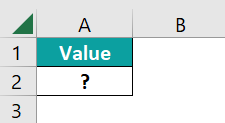The procedure to find the value using ISLOGICAL formula is,

Select cell B2, enter the formula =ISLOGICAL(abc), and press the “Enter” key.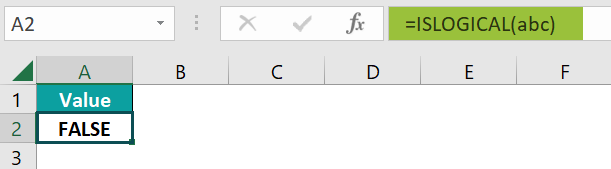The result is “FALSE”, as shown above.

2. How to insert the ISLOGICAL Excel function?

We can insert the ISLOGICAL Excel function as follows:
1. Select an empty cell for the output.
2. Type =ISLOGICAL( in the selected cell. [Alternatively, type =I or =IS and double-click the ISLOGICAL function from the list of suggestions shown by Excel.]
3. Enter the arguments as cell values or cell references and close the brackets.
4. Press the “Enter” key.

For example, we will find the logical value cells for the results of formulas using ISLOGICAL function.

In the table, the data is,
Column A contains the Value.
Column B contains the Output.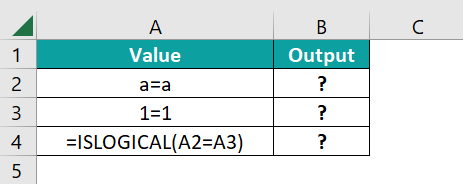The steps to find the value using ISLOGICAL formula are,

• Step 1: Select cell B2, enter the formula =ISLOGICAL(A2), and press the “Enter” key. The result is “FALSE”, as shown below.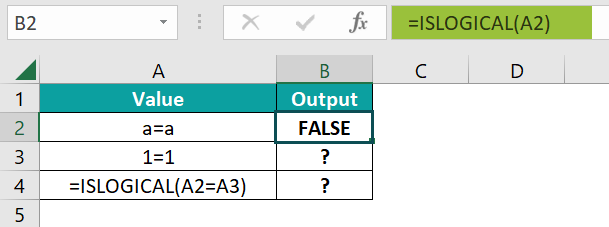• Step 2: Drag the formula from cell B2 to B4 using the fill handle. The output is shown below.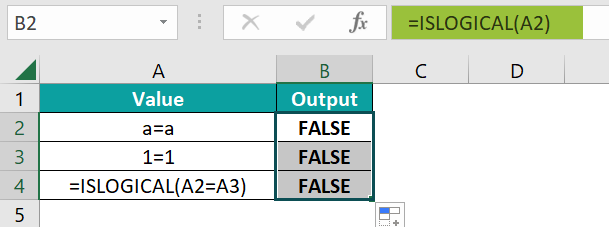3. Where is the ISLOGICAL Excel function?

The ISLOGICAL Excel function is in the “Formulas” tab, as shown below.

Choose an empty cell for the output → select the “Formulas” tab → go to the “Function Library” group → click the “More Functions” option drop-down → click the “Information” option right arrow → select the “ISLOGICAL” function, as shown below.